Geometric progression

Fill 4 numbers between 4 and -12500 to form geometric progression.

Result

a1:  -20
a2:  100
a3:  -500
a4:  2500

Solution:Leave us a comment of example and its solution (i.e. if it is still somewhat unclear...):Be the first to comment!Next similar examples:

1. SequenceFind the common ratio of the sequence -3, -1.5, -0.75, -0.375, -0.1875. Ratio write as decimal number rounded to tenth.
2. Geometric progression 48,4√2,4,2√2
3. Gp - 80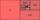Sum of the first four members of a geometric progression is 80. Determine they if we know that the fourth member is nine times greater than the second.
4. GP membersThe geometric sequence has 10 members. The last two members are 2 and -1. Which member is -1/16?
5. Six termsFind the first six terms of the sequence a1 = -3, an = 2 * an-1
6. Geometric progression 2There is geometric sequence with a1=5.7 and quotient q=-2.5. Calculate a17.
7. Piano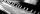If Suzan practicing 10 minutes at Monday; every other day she wants to practice 2 times as much as the previous day, how many hours and minutes will have to practice on Friday?
8. Geometric sequence 4It is given geometric sequence a3 = 7 and a12 = 3. Calculate s23 (= sum of the first 23 members of the sequence).
9. GP - 8 itemsDetermine the first eight members of a geometric progression if a9=512, q=2
10. A perineumA perineum string is 10% shorter than its original string. The first string is 24, what is the 9th string or term?
11. Five membersWrite first 5 members geometric sequence and determine whether it is increasing or decreasing: a1 = 3 q = -2
12. Tenth memberCalculate the tenth member of geometric sequence when given: a1=1/2 and q=2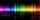The glass of 1 mm thickness absorb 4% of UV radiation passing through. How many percent of UV rays absorb glass with a thickness 1.1 cm, made from 1 mm thick those glasses?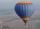Hot air balloon ascends 25 meters up for a minute after launch. Every minute ascends 75 percent of the height which climbed in the previous minute. a) how many meters ascends six minutes after takeoff? b) what is the overall height 10 minutes after launchWe want to prove the sentence: If the natural number n is divisible by six, then n is divisible by three. From what assumption we started?How much is sum of square root of six and the square root of 225?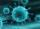We have a virus that lives one hour. Every half hour produce two child viruses. What will be the living population of the virus after 3.5 hours?